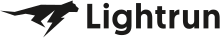• Dev Observability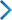• Product• Pricing
• Docs
• Resources• Blog
• Company• Debug Wordle# Stuck on an issue?

Lightrun Answers was designed to reduce the constant googling that comes with debugging 3rd party libraries. It collects links to all the places you might be looking at while hunting down a tough bug.

And, if you’re still stuck at the end, we’re happy to hop on a call to see how we can help out.

## "colour.HSL_to_RGB" definition provides incorrect RGB values for S = 1.

See original GitHub issue

### Issue Description

When one creates an array of hsl values `HSL = numpy.array([[0, 0, .5], [0, 1, .5]])`, and then one converts HSL to RGB using `RGB = colour.HSL_to_RGB(HSL)` one finds that `RGB = RGB`.

This should not be the case, as it should be: `RGB = array([1, 0, 0])`, while the package gives back: `RGB = array([0.5, 0.5, 0.5])`

If one creates an HSL hue-page, as shown with the problem above, the problem is clearly demonstrated as simply this package reverts values of S = 1 to S = 0.

I created a function to create and save HSL hue-pages through the process below:

``````import numpy as np
import colour
from PIL import Image as im

def plot_HSL_huepage(hue):
s = np.arange(0, 100.1, .1) / 100
l = np.arange(0, 100.1, .1) / 100
mesh = np.array(np.meshgrid(s, l))
combinations = mesh.T.reshape(-1, 2)
h = np.full((combinations.shape), hue)/360
hsl = np.stack([h, combinations[:,0], combinations[:,1]], axis = -1)
srgb = colour.HSL_to_RGB(hsl)
srgb = (srgb*255).astype(np.uint8)
RGB = np.reshape(srgb, (s.size, l.size, 3))
data = im.fromarray(RGB)
data = data.rotate(90)
data.save('hsl_huepage_h={}.png'.format(hue))
``````

When one then runs the function for HSL hues of 0, 60, 120, 240, and 300 as shown below:

``````h_values = np.array([0, 60, 120, 240, 300])
for x in h_values:
plot_HSL_huepage(x)
``````

when one opens the hue page png, no matter the hue, one sees that the values for S = 1 are the same grey values as S = 0.

I hope this issue submission is clear, I have never written one before. I only learned python because I wanted to use this package, and I am grateful for the amount of work put into making this package.

What can I do to get the correct RGB values using the `HSL_to_RGB` function?

### Issue Analytics

• State: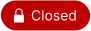• Created 2 years ago

####Top GitHub Comments

1reaction
KelSolaarcommented, Nov 2, 2020

Ah! Thanks for the kind words @nadersadoughi and glad to have people like you on-board! A key motivation for Colour was to democratize colour science and have it escape the Matlab sandbox! 😃

1reaction

@KelSolaar In fact, thank you for very quickly finding the solution! This is the very reason why I switched over from Matlab color packages to yours in python, because of this open-source community where we can make color better, together.

####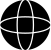Top Results From Across the Web

HSL to RGB color conversion problems - Stack Overflow
I replaced H, S, L values with my own names, hue, saturation, and luminosity. I looked it back over, but unless I am...
[css-color-3] HSL examples show incorrect RGB values #3088
HSL color values provides an algorithm for translating HSL to RGB, and states that the tables in section 4.2.4.1. HSL examples are generated ......
CSS Color Module Level 4 - W3C
CSS has several syntaxes for specifying color values: the sRGB hex color notation which represents the RGB and alpha channels in hexadecimal ...
HSL and HSV - Wikipedia
HSL (for hue, saturation, lightness) and HSV are alternative representations of the RGB color model, designed in the 1970s by computer graphics researchers ......
Converting Color Spaces in JavaScript - CSS-Tricks
It'll strip the % s and turn what's left into values out of 255. function RGBToHex(rgb) { let sep = rgb.indexOf(",") > -1...

####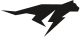Troubleshoot Live Code

Lightrun enables developers to add logs, metrics and snapshots to live code - no restarts or redeploys required.
Start Free

####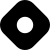Top Related Hashnode Post